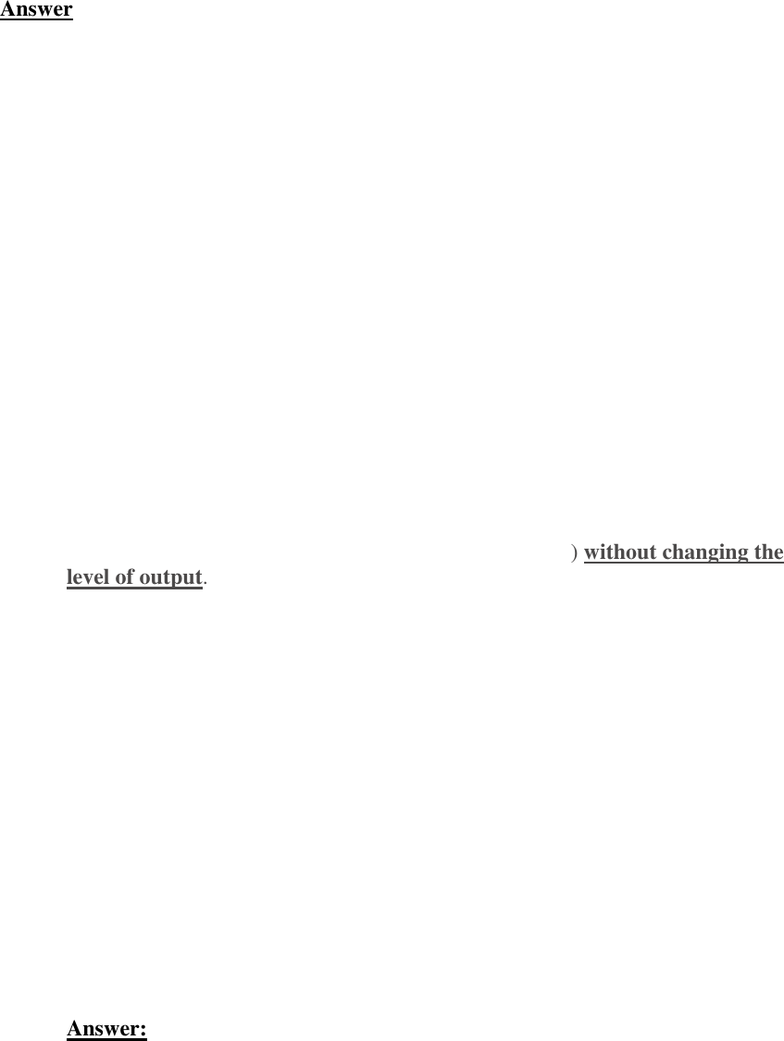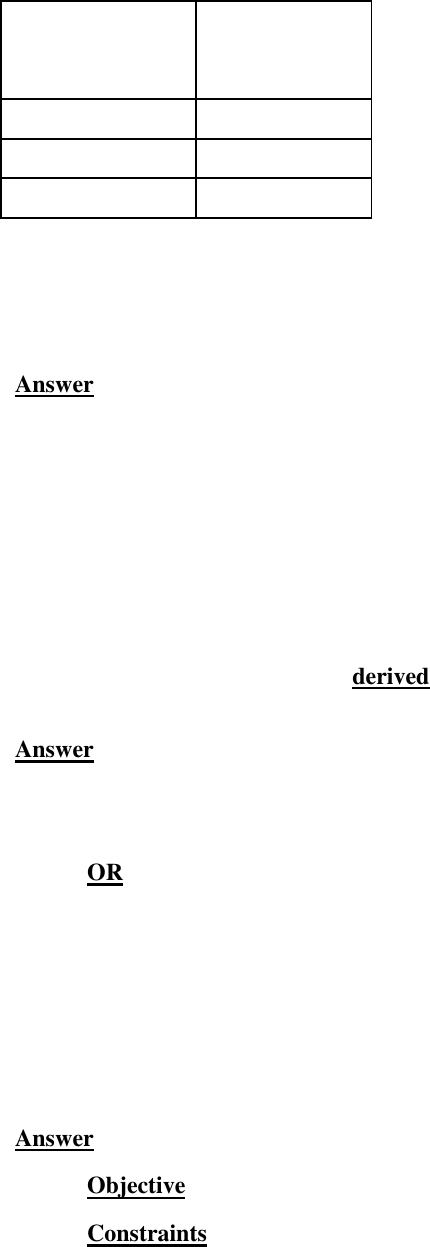Study Guides (390,000)
CA (150,000)
UOttawa (10,000)
ECO (300)
Midterm

ECO 2114 Study Guide - Midterm Guide: Marginal Revenue Productivity Theory Of Wages, Marginal Revenue, Marginal ProductExam

Department
Economics
Course Code
ECO 2114
Professor
David Gray
Study Guide
Midterm

This preview shows pages 1-3. to view the full 9 pages of the document.1
ECO 2114A MIDTERM EXAM II TIME: 1 hour and 45 minutes Nov 19, 2018
Professor: M. Rafiquzzaman OUTLINE OF SOLUTION Fall 2018
in the booklet).
Multiple Choice Answers (2x12 = 24 points)
1. C
2. B
3. A
4. D
5. C
6. A
7. C
8. B
9. C
10. D
11. D
12. B
booklet. Show your calculations as required. USE PEN)
PLEASE NOTE THAT GRAPHS ARE UPLOADED IN A SEPARATE FILE:
MIDTDERM 1 GRAPHS
1. Define (4 x 2 = 8 points)
a) Marginal revenue product
b) Value of the marginal physical product
c) Marginal rate of technical substitution
d) Wage elasticity of demand for labour

Only pages 1-3 are available for preview. Some parts have been intentionally blurred.2
a) Marginal revenue product
The marginal revenue product of an input (labour) is defined as: the marginal
revenue of output (MRQ) times the marginal physical product of the input
(labour). That is,
MRPL = MRQ x MPPL
b) Value of the marginal physical product
The value of the marginal product of an input (labour) is defined as: the output
price (P) times the marginal physical product of the input (labour). That is,
VMPL = P x MPPL
c) Marginal rate of technical substitution
The marginal rate of technical substitution (MRTS) is the rate at which one input
(labour L) can be substituted for another input (capital K) without changing the
level of output.
MRTS (of labour for capital) may be written as: MRST = (MPL/MPK)
d) Wage elasticity of demand for labour
Wage elasticity of demand for labour = (% change in the quantity of labour
demanded) / % change in the wage rate.
2. The table below gives the wage schedule and the supply of labour
schedule for a worker.
a) Assume that the workers reservation wage is \$7 per hour. Draw the
workers supply curve for labour. (1 + 2 = 3 points)
Please see Figure 1 in the Graph File II

Only pages 1-3 are available for preview. Some parts have been intentionally blurred.3
Wage rate in
dollars (W)
Supply of
labour in hours
(h)
8
8
10
12
14
10
b) Is the supply curve backward bending? Why? Explain. (2 + 4 = 6 points)
Yes. (2 points)
The backward bending segment of the labour supply curve, that is, downward-
sloping segment of the labour supply curve implies that income effects are
stronger than the substitution effects (that is, income effects > the substitution
effects). (4 points)
3. The demand for labour is a derived demand. Why? explain. (2 points)
The demand for labour is a derived demand because it depends on the demand for
the produced and supplied goods and services.
OR
Labour demand is derived demand, that is, labour is hired in order to produce
goods that are sold in the product market. For this reason, labour demand is
always connected to the product market, especially in terms of the degree of
competition, whether from foreign or domestic competition.
4. Demand for labour depends on the firms objectives and constraints. Name the
objective and constraints. (1 + 5 = 6 points)